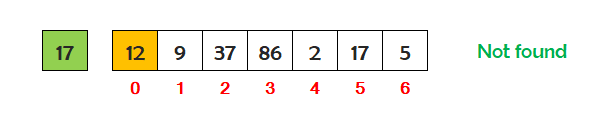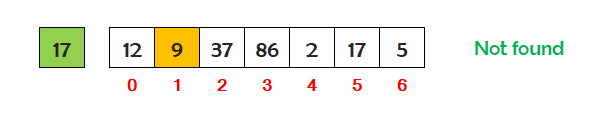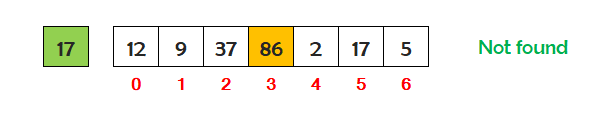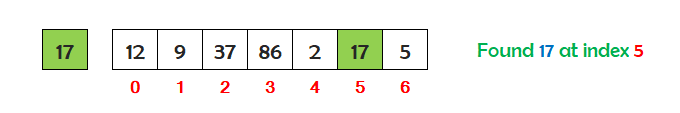# Linear Search in C Programming

###### Searching in C

In this lesson, we will understand what is Linear Search in C Programming along with some examples.

## What is Linear Search in C?

A Linear Search also known as Sequential Search is a searching technique used in C programming to search an element from an array in a linear fashion.

In this searching technique, an element is searched in sequential order one by one in an array from start to end. If the element is found, then the search is successful and, we print the position of the element. If not found, then the element does not exist in the array.

To clearly understand the linear search technique, let's go through its algorithm step by step with an example.## Linear Search Visualization

Suppose we have seven numbers stored in an array as shown below. We want to search the number 17 in the array.Take the 1st number 12 and compare it with the number 17. It's not matching so, check 17 with the next number.Now take the 2nd number 9 and compare it with the number 17. It's not matching so, check 17 with the next number.Now take the 3rd number 37 and compare it with the number 17. It's not matching so, check 17 with the next number.Now take the 4th number 86 and compare it with the number 17. It's not matching so, check 17 with the next number.Now take the 5th number 2 and compare it with the number 17. It's not matching so, check 17 with the next number.Now take the 6th number 17 and compare it with the number 17. It's matching so we, have found the number 17 at index 5 in the array.

## Algorithm for Linear Search

An algorithm is the steps used to implement the linear search in a c program.

Assume we have N numbers stored in an array. To implement the linear search on N numbers, the steps are as follows.

• Define an array to store N numbers for linear search. Suppose we have defined an array with the name num.
• Store the number we want to search in a variable say x.
• Declare a variable f and set its value 0. For example f=0.
• Run a loop i from 0 to N-1 to read each number from the array.
• Check if the value of x is equal to the value of num[i].
• If it is equal, then print the message Number found at index and the value of i on the screen and set the value of f to 1 and break the loop.
• Outside the body of the loop i check if value of f is 0 then print the message Number not found.

## C Program for Linear Search

A C program to search a number in an array using linear search technique.

#### Linear Search

``````#include <stdio.h>
#include <conio.h>

int main()
{
// define an array
int num[]= {12,9,37,86,2,17,5};
int i,x,f;

printf("Array: ");
for(i=0; i<7; i++)
{
printf("%d ",num[i]);
}

// store the number in variable x to search in the array
printf("\n\nEnter number to search ");
scanf("%d",&x);

// set the value of variable f=0
f=0;

// run loop i from 0 to N-1 to read each number from the array
for(i=0; i<7; i++)
{
// check if value of x is equal to the value of num[i] then print
// message "Number found at index" and the value of i and set f=1 and break the loop
if(x==num[i])
{
printf("Number found at index %d",i);
f=1;
break;
}
}

// check if value of f is 0 then print number not found
if(f==0)
{
}
return 0;
}``````

#### Output

```Array: 12 9 37 86 2 17 5

Enter number to search 17
Number found at index 5```

## Linear Search Program Explanation

The explanation of the above program is given below in details.

#### Explanation

```Array: 12 9 37 86 2 17 5

Number to be searched in the array
x = 17
f = 0
The loop i start running from 0 to 6

When i = 0
Value of x=17 and num=12
Check if 17==12  No

When i = 1
Value of x=17 and num=9
Check if 17==9  No

When i = 2
Value of x=17 and num=37
Check if 17==37  No

When i = 3
Value of x=17 and num=86
Check if 17==86  No

When i = 4
Value of x=17 and num=2
Check if 17==2  No

When i = 5
Value of x=17 and num=17
Check if 17==17  Yes
Number found at index 5

f = 1
Break the loop
Loop i ends

Check if f==0   No```